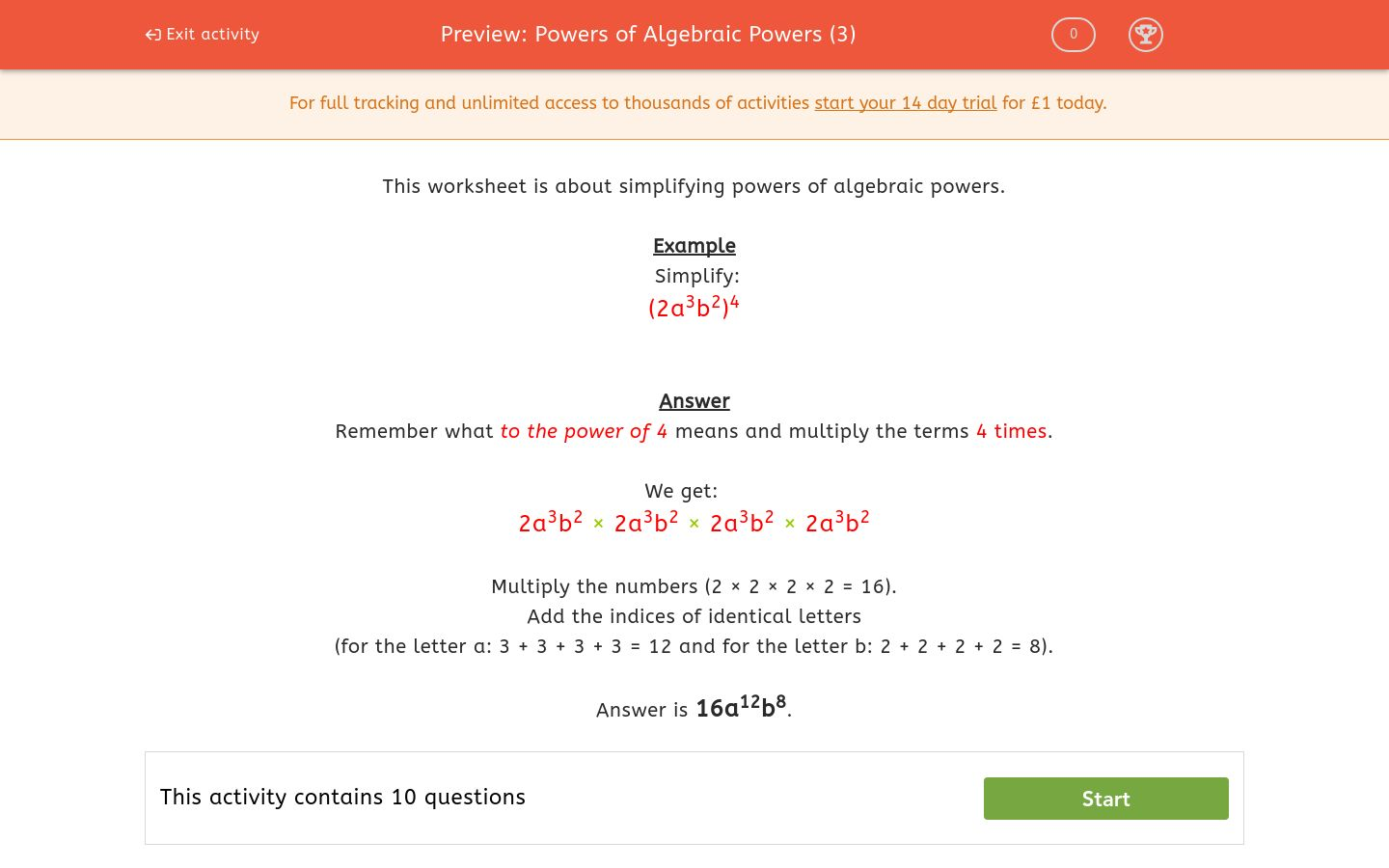# Powers of Algebraic Powers (3)

In this worksheet, students must simplify powers of algebraic powers.Key stage:  KS 3

Curriculum topic:  Algebra

Curriculum subtopic:  Simplify Algebraic Expressions to Maintain Equivalence

Difficulty level:### QUESTION 1 of 10

This worksheet is about simplifying powers of algebraic powers.

Example

Simplify:

(2a3b2)4

Remember what to the power of 4 means and multiply the terms 4 times.

We get:

2a3b2 × 2a3b2 × 2a3b2 × 2a3b2

Multiply the numbers (2 × 2 × 2 × 2 = 16).

Add the indices of identical letters

(for the letter a: 3 + 3 + 3 + 3 = 12 and for the letter b: 2 + 2 + 2 + 2 = 8).

Simplify:

(2a3b)2

4a32b2

4a5b

4a6b2

Simplify:

(3a2b2)2

6a22b22

9a4b4

6a4b4

Simplify:

(3a3b2)2

9a32b22

9a6b4

9a6b2

Simplify:

(5ab2)2

10ab4

25a2b4

10ab2

Simplify:

(3cb2)3

27c3b6

27c3b5

9c3b6

Simplify:

(2c3d2)3

8c3d6

8c6d5

8c9d6

Simplify:

(2f3d2)4

8f7d6

16f6d5

16f12d8

Simplify:

(3f3g)4

3f7g4

81f12g4

27f12g

Simplify:

(2p3q)6

64p18q6

12p9q6

12p18q

Simplify:

(2p3q4)7

128p10q11

128p21q11

128p21q28

• Question 1

Simplify:

(2a3b)2

4a6b2
EDDIE SAYS
2a3b × 2a3b
2 × 2 × a3 × a3 × b × b
• Question 2

Simplify:

(3a2b2)2

9a4b4
EDDIE SAYS
3a2b2 × 3a2b2
3 × 3 × a2 × a2 × b2 × b2
• Question 3

Simplify:

(3a3b2)2

9a6b4
EDDIE SAYS
3a3b2 × 3a3b2
3 × 3 × a3 × a3 × b2 × b2
• Question 4

Simplify:

(5ab2)2

25a2b4
EDDIE SAYS
5ab2 × 5ab2
5 × 5 × a × a × b2 × b2
• Question 5

Simplify:

(3cb2)3

27c3b6
EDDIE SAYS
3cb2 × 3cb2 × 3cb2
3 × 3 × 3 × c × c × c × b2 × b2 × b2
• Question 6

Simplify:

(2c3d2)3

8c9d6
EDDIE SAYS
2c3d2 × 2c3d2 × 2c3d2
2 × 2 × 2 × c3 × c3 × c3 × d2 × d2 × d2
• Question 7

Simplify:

(2f3d2)4

16f12d8
EDDIE SAYS
2f3d2 × 2f3d2 × 2f3d2 × 2f3d2
2 × 2 × 2 × 2 × f3 × f3 × f3 × f3 × d2 × d2 × d2 × d2
• Question 8

Simplify:

(3f3g)4

81f12g4
EDDIE SAYS
3f3g × 3f3g × 3f3g × 3f3g
3 × 3 × 3 × 3 × f3 × f3 × f3 × f3 × g × g × g × g
• Question 9

Simplify:

(2p3q)6

64p18q6
EDDIE SAYS
2p3q × 2p3q × 2p3q × 2p3q × 2p3q × 2p3q
2 × 2 × 2 × 2 × 2 × 2 × p3 × p3 × p3 × p3 × p3 × p3 × q × q × q × q × q × q
• Question 10

Simplify:

(2p3q4)7